# How to Subtract Two Columns in Pandas DataFrame?

• Last Updated : 19 Dec, 2021

In this article, we will discuss how to subtract two columns in pandas dataframe in Python.

Dataframe in use: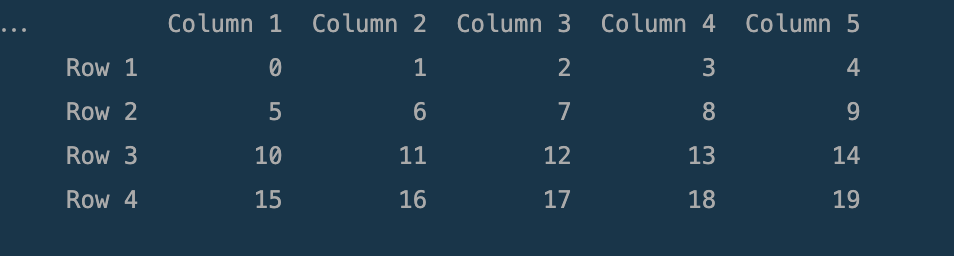## Method 1: Direct Method

This is the __getitem__ method syntax ([]), which lets you directly access the columns of the data frame using the column name.

Example: Subtract two columns in Pandas dataframe

## Python3

 `import` `numpy as np``import` `pandas as pd`` ` `data ``=` `np.arange(``0``, ``20``).reshape(``4``, ``5``)`` ` ` ` `df1 ``=` `pd.DataFrame(data,``                   ``index``=``[``'Row 1'``, ``'Row 2'``, ``'Row 3'``, ``'Row 4'``],``                   ``columns``=``[``'Column 1'``, ``'Column 2'``, ``'Column 3'``,``                            ``'Column 4'``, ``'Column 5'``])`` ` `# using our previous example``# now let's subtract the values of two columns``df1[``'Column 1'``] ``-` `df1[``'Column 2'``]`

Output: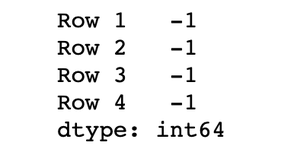## Method 2: Defining a function

We can create a function specifically for subtracting the columns, by taking column data as arguments and then using the apply method to apply it to all the data points throughout the column.

Example: Subtract two columns in Pandas dataframe

## Python3

 `import` `numpy as np``import` `pandas as pd`` ` `def` `diff(a, b):``    ``return` `b ``-` `a`` ` `data ``=` `np.arange(``0``, ``20``).reshape(``4``, ``5``)`` ` ` ` `df ``=` `pd.DataFrame(data,``                  ``index``=``[``'Row 1'``, ``'Row 2'``, ``'Row 3'``, ``'Row 4'``],``                  ``columns``=``[``'Column 1'``, ``'Column 2'``, ``'Column 3'``,``                           ``'Column 4'``, ``'Column 5'``])`` ` ` ` `df[``'Difference_2_1'``] ``=` `df.``apply``(``    ``lambda` `x: diff(x[``'Column 2'``], x[``'Column 2'``]), axis``=``1``)`

Output :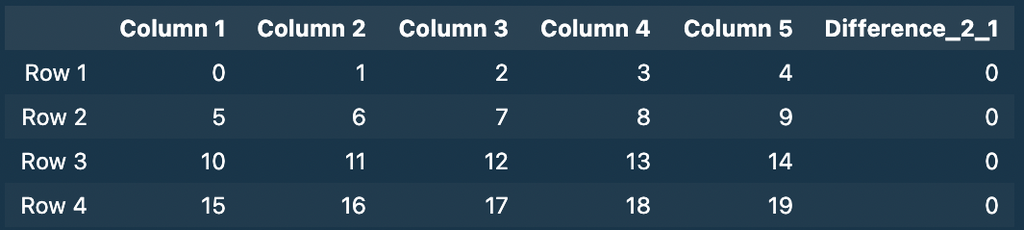## Method 3: Using apply()

Since the operation we want to perform is simple we can you can directly use the apply() method without explicitly defining a function. Provide the axis argument as 1 to access the columns.

Syntax:

s.apply(func, convert_dtype=True, args=())

Parameters:

• func: .apply takes a function and applies it to all values of pandas series.
• convert_dtype: Convert dtype as per the function’s operation.

Return Type: Pandas Series after applied function/operation.

Example: Subtract two columns in Pandas Dataframe

## Python3

 `import` `pandas as pd``import` `numpy as np`` ` `data ``=` `np.arange(``0``, ``20``).reshape(``4``, ``5``)`` ` ` ` `df ``=` `pd.DataFrame(data,``                  ``index``=``[``'Row 1'``, ``'Row 2'``, ``'Row 3'``, ``'Row 4'``],``                  ``columns``=``[``'Column 1'``, ``'Column 2'``, ``'Column 3'``,``                           ``'Column 4'``, ``'Column 5'``])`` ` ` ` `df[``'diff_3_4'``] ``=` `df.``apply``(``lambda` `x: x[``'Column 3'``] ``-` `x[``'Column 4'``], axis``=``1``)``df`

Output: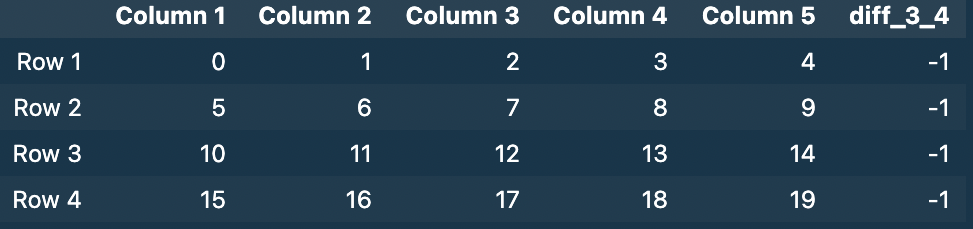## Method 4: Using the Assign method

assign() method assign new columns to a DataFrame, returning a new object (a copy) with the new columns added to the original ones.

Example: Subtract two columns in Pandas dataframe

## Python3

 `import` `numpy as np``import` `pandas as pd`` ` `data ``=` `np.arange(``0``, ``20``).reshape(``4``, ``5``)`` ` ` ` `df ``=` `pd.DataFrame(data,``                  ``index``=``[``'Row 1'``, ``'Row 2'``, ``'Row 3'``, ``'Row 4'``],``                  ``columns``=``[``'Column 1'``, ``'Column 2'``, ``'Column 3'``,``                           ``'Column 4'``, ``'Column 5'``])`` ` ` ` `df ``=` `df.assign(diff_1_5``=``df[``'Column 1'``] ``-` `df[``'Column 5'``])`` ` `df`

Output :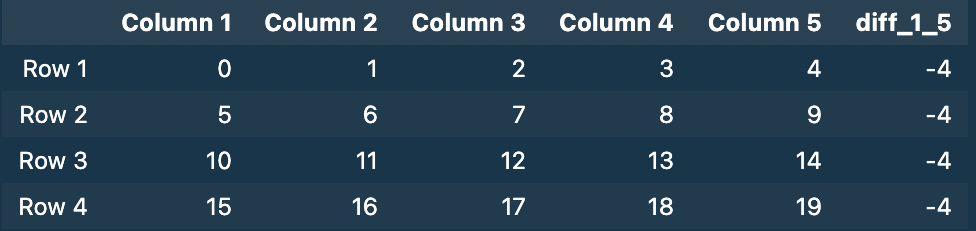My Personal Notes arrow_drop_up